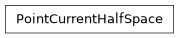# geoana.em.static.PointCurrentHalfSpace#class geoana.em.static.PointCurrentHalfSpace(rho, current=1.0, location=None)#

Class for a point current in a halfspace.

The PointCurrentHalfSpace class is used to analytically compute the potentials, current densities and electric fields within a halfspace due to a point current. Surface is assumed to be at z=0.

Parameters
currentfloat

Electrical current in the point current (A). Default is 1A.

rhofloat

Resistivity in the point current ($$\Omega \cdot m$$).

locationarray_like, optional

Location at which we are observing in 3D space (m). Default is (0, 0, 0).

Attributes

 current Current in the point current in Amps. location Location of observer in 3D space. rho Resistivity in the point current in $$\Omega \cdot m$$.

Methods

 Current density for a point current in a halfspace. Electric field for a point current in a halfspace. potential(xyz) Electric potential for a point current in a halfspace.